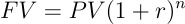← Back to Glossary

# Future Value

Based on an expected growth rate, future value (FV) is the value of a current investment at a specific point in time in the future based on an assumed growth rate. The future value calculation enables investors to understand whether an investment is worthwhile, determine if there are better investments over time, and define what the potential value of their current holdings might be in the future.

The future value of any investment depends on a wide variety of factors, including the type of investment, the periodic interest rate, the expected rate of return, and the length of the investment.

The future value formula helps business leaders make rational decisions about how much they want to save each month and what percentage of their income they want to invest over a particular time period.

## Calculating the future value

Depending on the type of asset, it will be more difficult to calculate its future value, as this is directly related to the stability or volatility of its growth rate.

In any case, there are two ways to calculate the future value of an asset: by using a simple annual interest rate or by using a compound annual interest rate.

The main distinction is that the compound annual interest technique applies an interest rate to the cumulative account balance for each period, whereas the simple annual interest approach assumes a constant growth rate and a single initial payment, in which the interest rate is earned.

## The pros & cons of the future value calculation

One of the main reasons why the future value formula is so popular is because it helps investors make more effective business decisions as it allows for planning and gives them insight into the future. Which is particularly useful in uncertain market conditions.

Moreover, it lets them compare different investment options by contrasting their present value with their expected value. As it is based on estimates, this is a very easy metric for investors to calculate to assume an investment profit.

The future value is a common indicator among companies because it provides a simple way to think about how much money they could make over a certain period of time. However, there are several disadvantages to using it.

First, it usually assumes a stable rate of growth, which may not always be realistic as growth tends to fluctuate from one year to the next. Second, it relies on some specific assumptions that might never occur. Lastly, it may become a complex indicator to calculate annuities or cash flow irregularities.## Related Content

No items found.